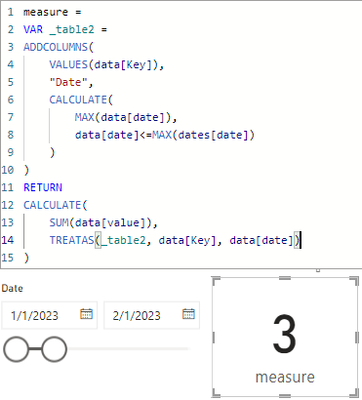cancel
Showing results for
Did you mean:Regular Visitor

## How to calculate sum of values by last date and distinct key

Hi!
I have a problem where I need to calculate sum (and average) of values, by only using the last applicable date (filtered by date-filter), and only using distinct keys related to the values.

Example of data:

 Key Value Date a 1 1.1.2023 a 2 2.1.2023 a 3 3.1.2023 b 3 10.2.2023 b 2 20.2.2023 b 1 10.5.2023 c 5 5.2.2023 c 5 6.2.2023 c 5 23.5.2023

Dates can be very randomly distributed (based on modification of data).

For example if user filter date = 1.3.2023, applicable rows would be: (last rows before that point of time)

 a 3 3.1.2023
 b 2 20.2.2023
 c 5 6.2.2023

Which would return 8 for sum.

I couldn't find a case with such complexity, only simpler sumx distinct examples, but I also need to dynamically consider which is the last applicable date by that point of time.

Thank you

Antti Järvinen

1 ACCEPTED SOLUTIONCommunity Champion

not sure if i fully get you. try to

1) plot a slicer with a calculated table like:

``dates = CALENDAR(MIN(data[Date]), MAX(data[Date]))``

2) plot a measure like:

``````measure =
VAR _table2 =
VALUES(data[Key]),
"Date",
CALCULATE(
MAX(data[date]),
data[date]<=MAX(dates[date])
)
)
RETURN
CALCULATE(
SUM(data[value]),
TREATAS(_table2, data[Key], data[date])
)``````

it worked like:Only a has a 3.a has 3, b as 2, c has 53 REPLIES 3Regular Visitor

I noticed a problem when I have a more complicated situation, where I need to apply more filters than just the Date, and to do this inside RETURN-clause (exclude filters from _table2).

==> I was able to solve this by creating a new calculated column which tracked the next Date-value. Therefore I was able to specify the validity date-range for each row.Community Champion

not sure if i fully get you. try to

1) plot a slicer with a calculated table like:

``dates = CALENDAR(MIN(data[Date]), MAX(data[Date]))``

2) plot a measure like:

``````measure =
VAR _table2 =
VALUES(data[Key]),
"Date",
CALCULATE(
MAX(data[date]),
data[date]<=MAX(dates[date])
)
)
RETURN
CALCULATE(
SUM(data[value]),
TREATAS(_table2, data[Key], data[date])
)``````

it worked like:Only a has a 3.a has 3, b as 2, c has 5Regular Visitor

Thank you, your idea works with small modifications to my use case, and therefore I accepted it as solution 🙂
(I didn't remember to specify in my original post that I need to plot the result of this function (y) against date (x), and so I further had to remove the relationship between data and calendar tables to make your solution work).
Otherwise, I think I would need to use e.g. ALL function somehow.. maybe you have an idea how to further modify the DAX-function to not be required to remove the relationship?